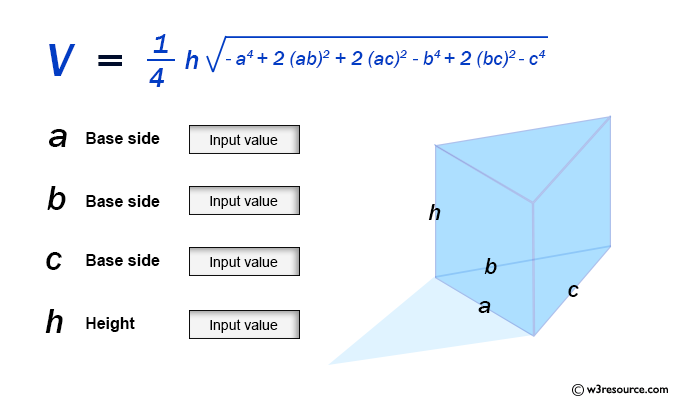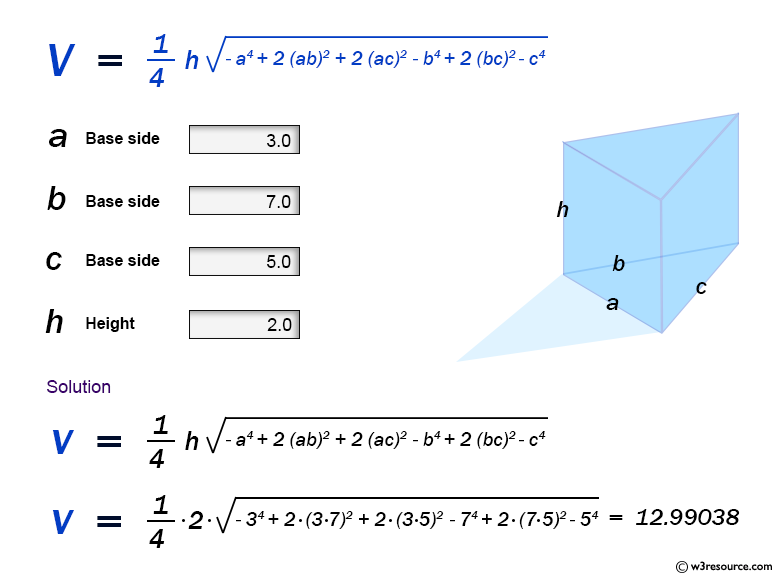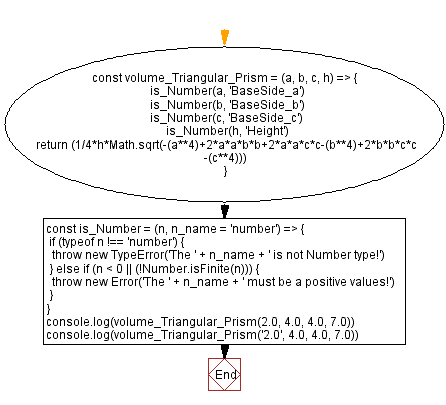# JavaScript: Volume of a Triangular Prism

## JavaScript Math: Exercise-61 with Solution

Write a JavaScript program to calculate the volume of a Triangular Prism.

From Wikipedia -
In geometry, a triangular prism is a three-sided prism; it is a polyhedron made of a triangular base, a translated copy, and 3 faces joining corresponding sides. A right triangular prism has rectangular sides, otherwise it is oblique. A uniform triangular prism is a right triangular prism with equilateral bases, and square sides.

Sample Data:Sample Solution:

HTML Code:

``````<!DOCTYPE html>
<html>
<meta charset="utf-8">
<title>JavaScript function to Volume of a Triangular Prism</title>
<body>

</body>
</html>
```
```

JavaScript Code:

``````const volume_Triangular_Prism = (a, b, c, h) => {
is_Number(a, 'BaseSide_a')
is_Number(b, 'BaseSide_b')
is_Number(c, 'BaseSide_c')
is_Number(h, 'Height')
return (1/4*h*Math.sqrt(-(a**4)+2*a*a*b*b+2*a*a*c*c-(b**4)+2*b*b*c*c-(c**4)))
}
const is_Number = (n, n_name = 'number') => {
if (typeof n !== 'number') {
throw new TypeError('The ' + n_name + ' is not Number type!')
} else if (n < 0 || (!Number.isFinite(n))) {
throw new Error('The ' + n_name + ' must be a positive values!')
}
}
console.log(volume_Triangular_Prism(2.0, 4.0, 4.0, 7.0))
console.log(volume_Triangular_Prism('2.0', 4.0, 4.0, 7.0))
```
```

Sample Output:

```27.11088342345192
-----------------------------------------------------------
Uncaught TypeError: The BaseSide_a is not Number type!
at https://cdpn.io/cpe/boomboom/pen.js?key=pen.js-d5ecc06d-a003-d50c-3d8f-00a52b5e6f98:10

```

Flowchart:Live Demo:

See the Pen javascript-math-exercise-61 by w3resource (@w3resource) on CodePen.

Improve this sample solution and post your code through Disqus

Previous: Volume of a Cylinder.
Next: Volume of a Pentagonal Prism

What is the difficulty level of this exercise?

Test your Programming skills with w3resource's quiz.

﻿